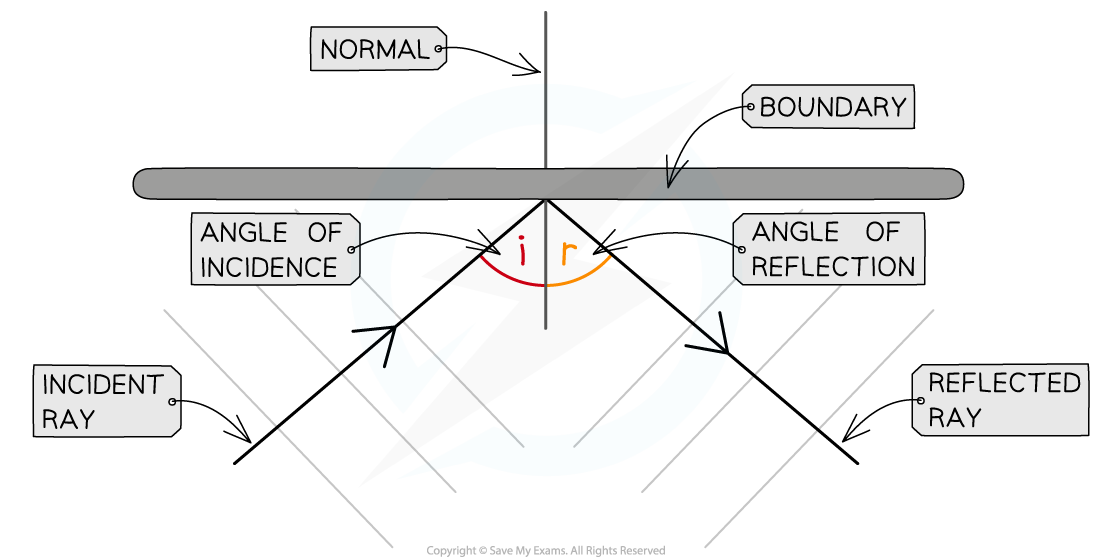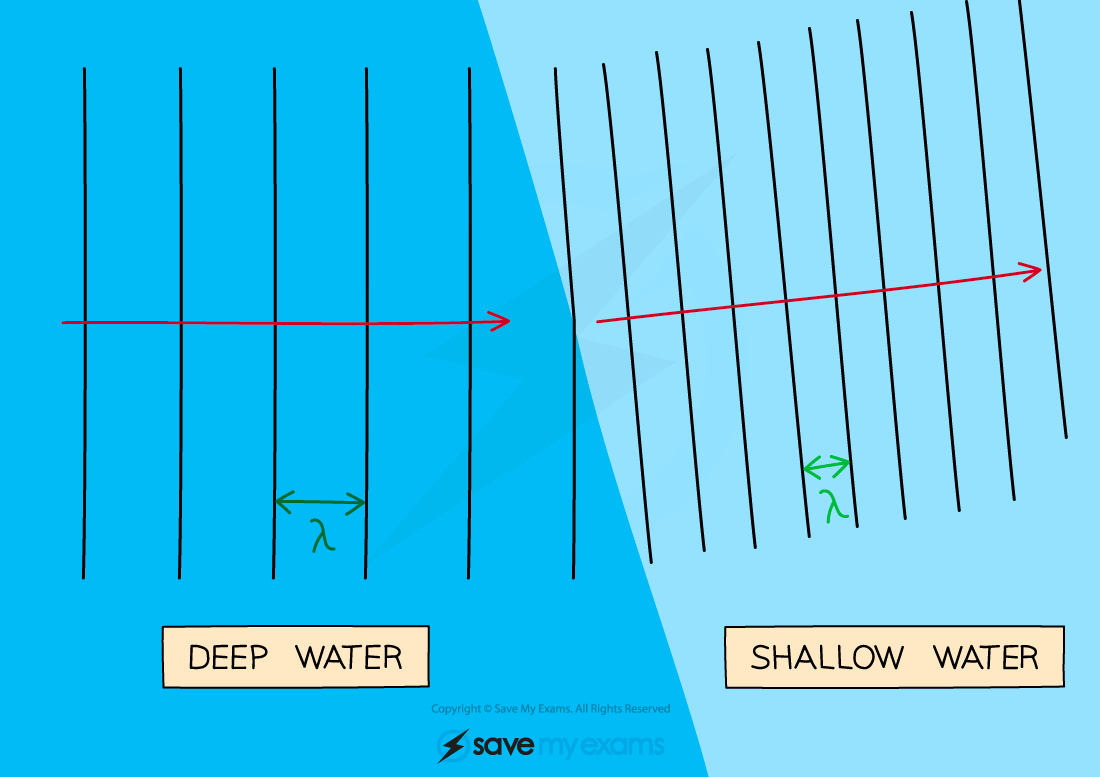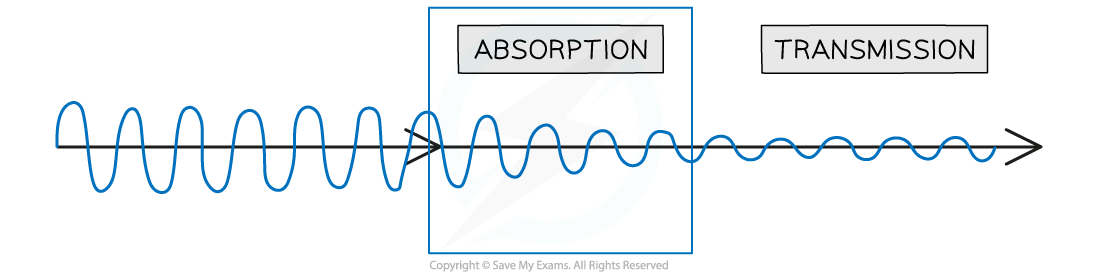# IB DP Physics: SL复习笔记4.4.1 Reflection, Refraction & Transmission

### Reflection, Refraction & Transmission

• When waves arrive at a boundary between two materials, they can be:
• Reflected
• Refracted
• Transmitted
• Absorbed

#### Reflection

• Reflection occurs when:

A wave hits a boundary between two media and does not pass through, but instead bounces back to the original medium

• The law of reflection states:

The angle of incidence, i = The angle of reflection, rReflection of a wave at a boundary

• When a wave is reflected, some of it may also be absorbed by the medium, transmitted through the medium, or polarised
• At a boundary between two media, the incident ray is the ray that travels towards the boundary

#### Refraction

• Refraction occurs when:

A wave changes speed and direction at the boundary between two media

• This is due to the density of the media
• If the medium is more dense, the wave slows down
• If the medium is less dense, the wave speeds up
• When a wave refracts, its speed and wavelength change, but its frequency remains the same
• This is noticeable by the fact that the colour of the wave does not change
• Both transverse and longitudinal waves can refract
• An example of water waves refracting is when they travel from deeper to shallower water
• The wavelength of the waves decrease in the shallower waterWater waves being refracted at the boundary between deep and shallow water

#### Transmission

• Transmission occurs when:

A wave passes through a substance

• Refraction is a type of transmission
• Transmission is the more general term of a wave appearing on the opposite side of a boundary (the opposite of reflection)
• Refraction is specifically the change in direction of a wave when it crosses a boundary between two materials that have a different density
•  When passing through a material, waves are usually partially absorbed
• The transmitted wave will have a lower amplitude if some absorption has occurredWhen a wave passes through a boundary it may be absorbed and transmitted